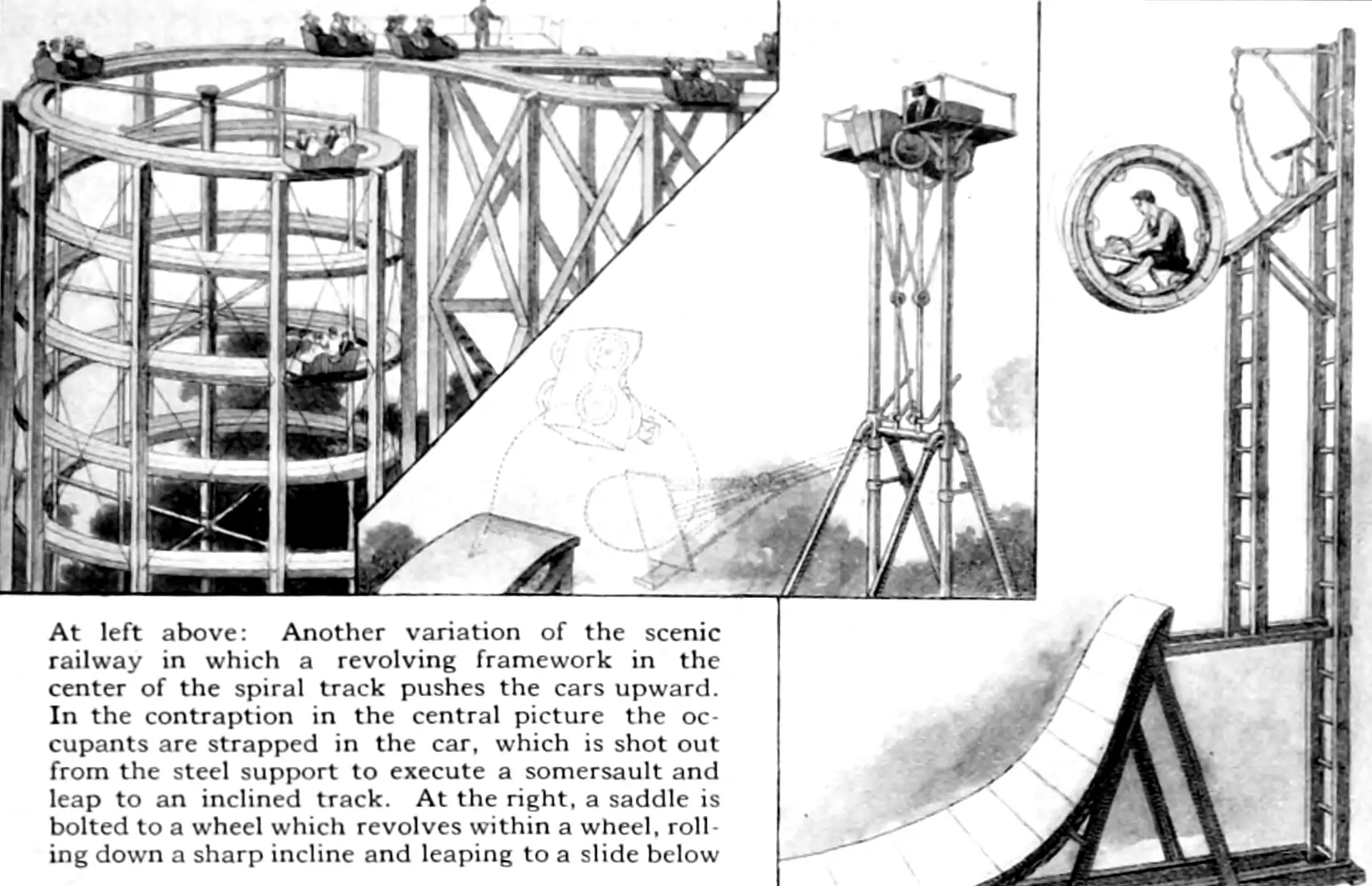# Subordinators

## Non-decreasing Lévy processes with weird branding

$\renewcommand{\var}{\operatorname{Var}} \renewcommand{\dd}{\mathrm{d}} \renewcommand{\pd}{\partial} \renewcommand{\bf}{\mathbf{#1}} \renewcommand{\vv}{\boldsymbol{#1}} \renewcommand{\rv}{\mathsf{#1}} \renewcommand{\mm}{\mathrm{#1}} \renewcommand{\cc}{\mathcal{#1}} \renewcommand{\oo}{\operatorname{#1}} \renewcommand{\gvn}{\mid} \renewcommand{\II}{\mathbb{I}}$

A subordinator is an a.s. non-decreasing Lévy process $$\{\rv{g}(t)\}, t \in \mathbb{R}$$ with state space $$\mathbb{R}_+\equiv [0,\infty]$$ such that

$\mathbb{P}(\rv{g}(t)-\rv{g}(s)< 0)=0, \,\forall t \geq s.$

That is, it is an a.s. non-decreasing Markov process with homogeneous independent increments.Superordinators

Tutorial introductions to these creatures are in .

The platonic ideal of a subordinator is probably the Lévy-Gamma process. However there are many more; There is no requirement that the support of a subordinator’s paths is dense in $$\mathbb{R}_+$$; for example, counting such Poisson processes are subordinators.

The terminology is weird. Why “subordinator”? Popular references mention their use as a model of random rate of passage of time in the popular Variance Gamma model . I know almost nothing about that but I read a little bit of history in . The idea is certainly older than that, though. Surely is implicit in Lamperti? I do not have time for that citation rabbit hole though.

The subordination of a one-dimensional Lévy process $$\{X(t)\}$$ by a one- dimensional increasing process $$\{T (t)\}$$ means introducing a new process $$\{Y (t)\}$$ defined as $$Y (t) = X(T (t))$$, where $$\{X(t)\}$$ and $$\{T (t)\}$$ are assumed to be independent, and that one-dimensional increasing process is the subordinator.

Subordinators can be generalised to beyond one-dimensional processes; see . We can more generally consider subordinators that take values in $$\mathbb{R}^d,$$ although such a process no longer has an intuitive interpretation as the rate-of-time-passing, since time is usually one-dimensional in common experience.

## PropertiesPure jump processes

Let us see what Dominic Yeo says, channelling Bertoin (1996):

In general, we describe Lévy processes by their characteristic exponent. As a subordinator takes values in $$[0,\infty),$$ we can use the Laplace exponent instead: $\mathbb{E}\exp(-\lambda X_t)=:\exp(-t\Phi(\lambda)).$ We can refine the Levy-Khintchine formula; $\Phi(\lambda)=k+d\lambda+\int_{[0,\infty)}(1-e^{-\lambda x})\Pi(dx),$ where k is the kill rate (in the non-strict case). Because the process is increasing, it must have bounded variation, and so the quadratic part vanishes, and we have a stronger condition on the Levy measure: $$\int(1\wedge x)\Pi(dx)<\infty.$$ The expression $$\bar{\Pi}(x):=k+\Pi((x,\infty))$$ for the tail of the Levy measure is often more useful in this setting. We can think of this decomposition as the sum of a drift, and a PPP with characteristic measure $$\Pi+k\delta_\infty.$$ … we do not want to consider the case that $$X$$ is a step process, so either $$d>0$$ or $$\Pi((0,\infty))=\infty$$ is enough to ensure this.

## Gamma processes

See Gamma processes.

## Beta processes

The strictly-increasing flavour, as seen in See Gamma processes.

## as measure priors

See Measure priors.

🏗

## Inverse Gaussian processes

See . Interesting because Minami (2003) gives an interpretation of the multivariate Inverse Gaussian process which is suggestive of a natural dependence model via covariance of a dual Gaussian process. Dependency structure might be interesting.

🏗

## An increasing linear function is a subordinator

We get linear functions both as a baked-in affordance of Lévy processes and as a limiting distribution of a Gamma process whose increment variance goes to zero.

## Positive linear combinations of other subordinators

Say we have a collection of $$m$$ independent univariate subordinators, not necessarily from the same family or with the same parameters, stacked to form a vector process $$\{\rv{g}(t)\}$$ with state space $$\mathbb{R}_+^{m}$$. Take a transform matrix $$M\in\mathbb{R}^{n\times m}$$ with non-negative entries. Then the process $$\{M\rv{g}(t)\}$$ is still a subordinator (and moreover a Lévy process) although the elements are no longer independent. We could think of that the same trick applied to just the increments, which would come down to the same thing.

🏗

## Subordination of subordinators

Since a subordinator time change of a Lévy process is still a Lévy process, a subordinated subordinator is still a Lévy process and it is still monotone and therefore still a subordinator. Is this convenient for my purposes?

🏗

## Generalized Gamma Convolutions

A construction that shows how to represent some startling (to me) processes using subordinators, including Pareto and Lognormal ones.

## via Kendall’s identity

leverage a neat result (Kendall’s identity) in to produce several interesting families of subordinators with explicit transition densities. These look handy.

## Multivariate

How do we construct a multivariate subordinator process, by which I mean a random process whose realisations are coordinate-wise monotone functions $$\mathbb{R}^D\to\mathbb{R}$$? One obvious way is to take coordinate-wise iid subordinators as the distributions of each coordinate. This is by construction what we want. Questions: How general is such a thing? How easy is it to conditionally sample paths from it? What do we want our multivariate subordinator to do? I care about this especially for multivariate Gamma processes.

### No comments yet. Why not leave one?

GitHub-flavored Markdown & a sane subset of HTML is supported.